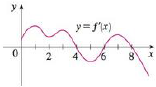Chapter 4.3, Problem 8E### Single Variable Calculus: Early Tr...

8th Edition
James Stewart
ISBN: 9781305270343

#### Solutions

Chapter
Section### Single Variable Calculus: Early Tr...

8th Edition
James Stewart
ISBN: 9781305270343
Textbook Problem

# The graph of the first derivative f′ of a function f is shown.(a) On what intervals is f increasing? Explain.(b) At what values of x does f have a local maximum or minimum? Explain.(c) On what intervals is f concave upward or concave downward? Explain.(d) What are the x-coordinates of the inflection points of f? Why?(a)

To determine

To explain: About the intervals of the given graph f where the function f is increasing.

Explanation

The function f(x) is increasing on the interval (a,b) if the first derivative of the given function is positive on (a,b) .

Since the given graph is the graph of the derivative function, and so from the given graph, it is observed that f(x)>0 on the intervals (0,4)

(b)

To determine

To explain: About the values of x where the function f have local maximum or local minimum.

(c)

To determine

To explain: About the intervals of the given graph f where the function f is concave upward or concave downward.

(d)

To determine

To find: The x-coordinates of the inflection point of the given function.

### Still sussing out bartleby?

Check out a sample textbook solution.

See a sample solution

#### The Solution to Your Study Problems

Bartleby provides explanations to thousands of textbook problems written by our experts, many with advanced degrees!

Get Started